These 10 Hacks Will Make You Seem Like A Math Genius

Looking for the best statistics problem solver or want to impress your friends with your jaw-dropping math skills? Here you’ll find the best math hacks you’ve:

1. How To Multiply By Elevenmath hack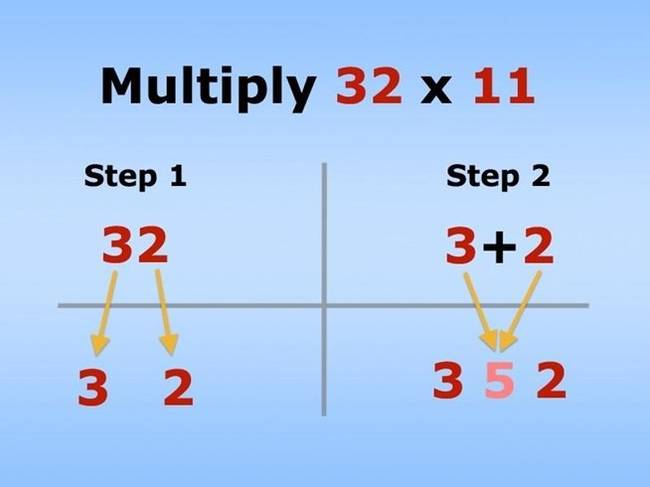2. How To Remember The First Eight Digits Of Pimath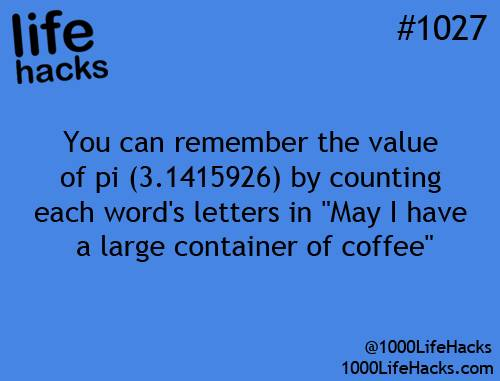3. Shortcut To Calculating Percentagesmath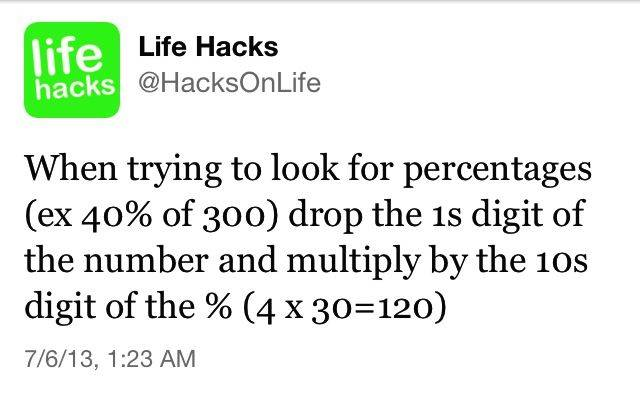4. Easy Way To Calculate Fractions Of Whole Numbersmath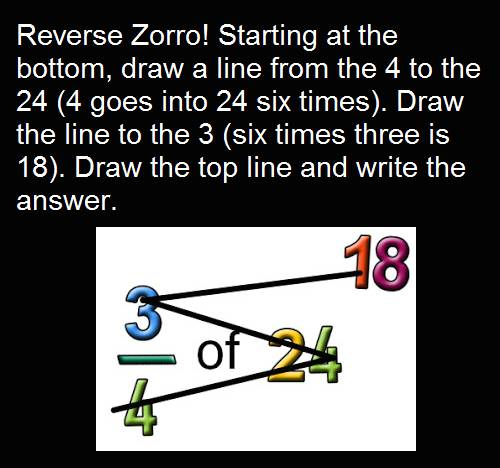5. How To Convert Celsius To Fahrenheitmath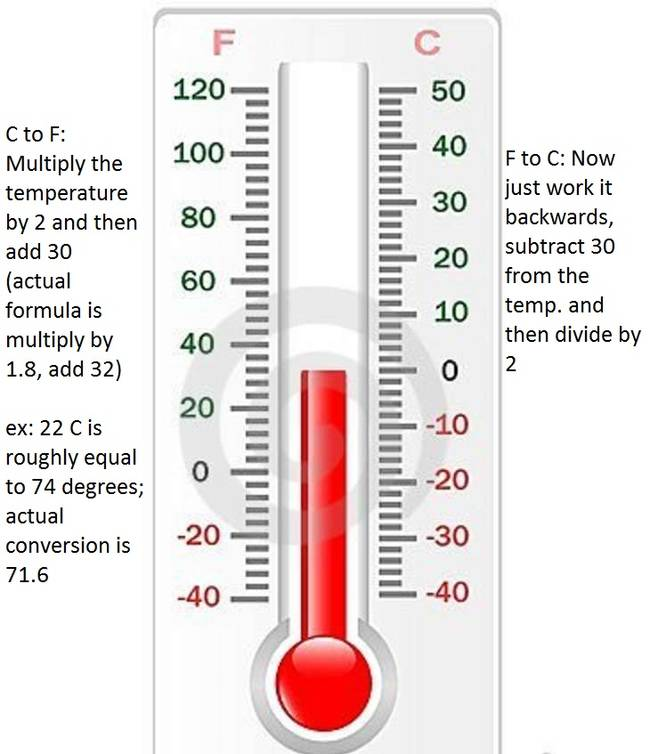6. Know Which Sign Means Greater Than And Which Means Less Thanmath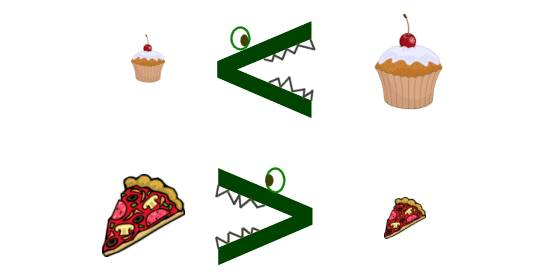7. Secret To Studying Mathmath hack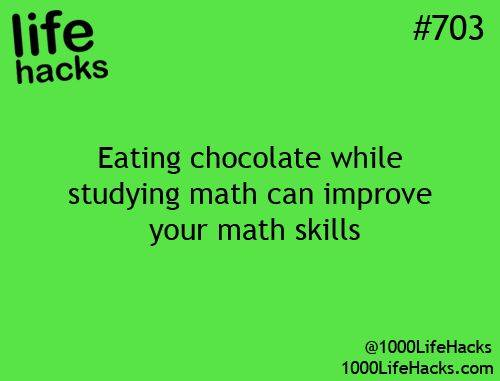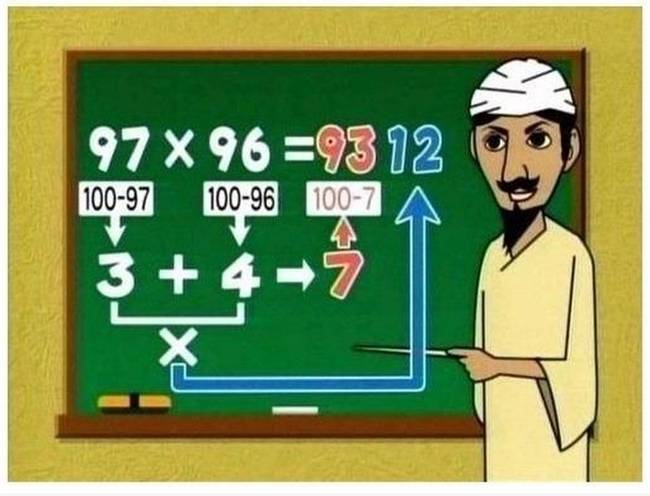9. Easy Way To Add And Subtract Fractionsmath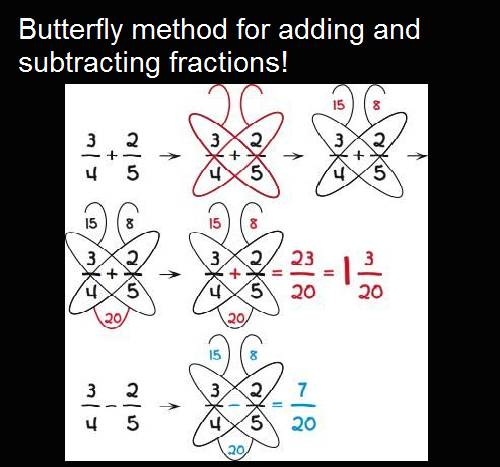1o. Multiples Of 9 Made Easymath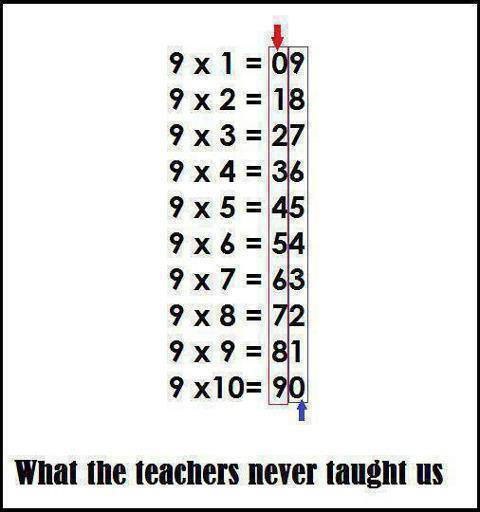VIA

Share the joy